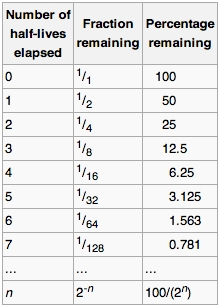## Half-Life

#### Learning Objective

• Distinguish between first-order, second-order, and zero-order half-life equations

#### Key Points

• The half-life equation for a first-order reaction is $t_{\frac{1}{2}}=\frac{ln(2)}{k}$ .
• The half-life equation for a second-order reaction is $t_{\frac{1}{2}}=\frac{1}{k[A]_{0}}$ .
• The half-life equation for a zero-order reaction is $t_{\frac{1}{2}}=\frac{[A]_{0}}{2k}$ .

#### Term

• half-lifeThe time required for a quantity to fall to half its value as measured at the beginning of the time period.

The half-life is the time required for a quantity to fall to half its initial value, as measured at the beginning of the time period. If we know the integrated rate laws, we can determine the half-lives for first-, second-, and zero-order reactions. For this discussion, we will focus on reactions with a single reactant.Half-lifeThe half-life of a reaction is the amount of time it takes for it to become half its quantity.

## Half-Life of a First-Order Reaction

Recall that for a first-order reaction, the integrated rate law is given by:

$[A]=[A]_0 e^{-(kt)}$

This can be written another way, equivalently:

$ln[A]=ln[A]_{0}-kt$

If we are interested in finding the half-life for this reaction, then we need to solve for the time at which the concentration, [A], is equal to half of what it was initially; that is, $\frac{[A]_0}{2}$. If we plug this in for [A] in our integrated rate law, we have:

$ln\frac{[A]_0}{2}=ln[A]_{0}-kt$

By rearranging this equation and using the properties of logarithms, we can find that, for a first order reaction:

$t_\frac{1}{2}=\frac{ln(2)}{k}$

What is interesting about this equation is that it tells us that the half-life of a first-order reaction does not depend on how much material we have at the start. It takes exactly the same amount of time for the reaction to proceed from all of the starting material to half of the starting material as it does to proceed from half of the starting material to one-fourth of the starting material. In each case, we halve the remaining material in a time equal to the constant half-life. Keep in mind that these conclusions are only valid for first-order reactions.

Consider, for example, a first-order reaction that has a rate constant of 5.00 s-1. To find the half-life of the reaction, we would simply plug 5.00 s-1 in for k:

$t_\frac{1}{2}=\frac{ln(2)}{k}$

$t_\frac{1}{2}=\frac{ln(2)}{5.00s^{-1}}=0.14\text{ s}$

## Half-Life for Second-Order Reactions

Recall our integrated rate law for a second-order reaction:

$\frac{1}{[A]}=\frac{1}{[A]_0}+kt$

To find the half-life, we once again plug in $\frac{[A]_0}{2}$for [A].

$\frac{1}{\frac{[A]_0}{2}}=\frac{1}{[A]_0}+kt$

$\frac{2}{[A]_0}=\frac{1}{[A]_0}+kt$

Solving for t, we get:

$t_\frac{1}{2}=\frac{1}{k[A]_0}$

Thus the half-life of a second-order reaction, unlike the half-life for a first-order reaction, does depend upon the initial concentration of A. Specifically, there is an inversely proportional relationship between $t_\frac{1}{2}$ and [A]0; as the initial concentration of A increases, the half-life decreases.

Consider, for example, a second-order reaction with a rate constant of 3 M-1 s-1 in which the initial concentration of A is 0.5 M:

$t_\frac{1}{2}=\frac{1}{(3)(0.5)}=0.67\text{ s}$

## Half-Life for a Zero-Order Reaction

The integrated rate law for a zero-order reaction is given by:

$[A]=[A]_0-kt$

Subbing in $\frac{[A]_0}{2}$ for [A], we have:

$\frac{[A]_0}{2}=[A]_0-kt$

Rearranging in terms of t, we can obtain an expression for the half-life:

$t_\frac{1}{2}=\frac{[A]_0}{2k}$

Therefore, for a zero-order reaction, half-life and initial concentration are directly proportional. As initial concentration increases, the half-life for the reaction gets longer and longer.